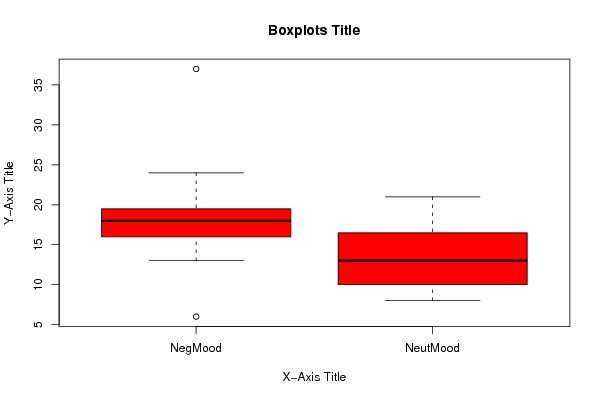Free Statistics

of Irreproducible Research!

Author's title
Author*The author of this computation has been verified*
R Software ModuleIan.Hollidayrwasp_Tests to Compare Two Means.wasp
Title produced by softwareAston University Statistical Software
Date of computationSun, 14 Mar 2010 13:49:38 +0100
Cite this page as followsStatistical Computations at FreeStatistics.org, Office for Research Development and Education, URL https://freestatistics.org/blog/index.php?v=date/2010/Mar/14/t12685712874n7044urjmq0q28.htm/, Retrieved Wed, 19 Jan 2022 09:58:21 +0000
Statistical Computations at FreeStatistics.org, Office for Research Development and Education, URL https://freestatistics.org/blog/index.php?pk=74380, Retrieved Wed, 19 Jan 2022 09:58:21 +0000
QR Codes:Original text written by user:
IsPrivate?No (this computation is public)
User-defined keywords
Estimated Impact137
Family? (F = Feedback message, R = changed R code, M = changed R Module, P = changed Parameters, D = changed Data)
-     [Aston University Statistical Software] [Morning Sickness ...] [2009-11-16 16:26:06] [74be16979710d4c4e7c6647856088456]
- R     [Aston University Statistical Software] [Morning Sickness ...] [2009-11-16 17:22:16] [74be16979710d4c4e7c6647856088456]
-   PD      [Aston University Statistical Software] [] [2010-03-14 12:49:38] [5b58052098f4b0433c56ae9d9a4453be] [Current]
-             [Aston University Statistical Software] [] [2010-03-14 12:56:02] [e8bb49267f0b4e611f4778412d0811f2]
-             [Aston University Statistical Software] [] [2010-03-14 12:56:02] [e8bb49267f0b4e611f4778412d0811f2]
-             [Aston University Statistical Software] [] [2010-03-14 12:59:30] [e8bb49267f0b4e611f4778412d0811f2]
-             [Aston University Statistical Software] [] [2010-03-14 13:01:10] [e8bb49267f0b4e611f4778412d0811f2]
Feedback Forum

Post a new message
Dataseries X:
6	8
24	10
21	12
18	10
16	17
37	10
16	8
18	16
18	16
13	18
22	21
16	13
18	8
16	14
14	18

 Summary of computational transaction Raw Input view raw input (R code) Raw Output view raw output of R engine Computing time 0 seconds R Server 'RServer@AstonUniversity' @ vre.aston.ac.uk

\begin{tabular}{lllllllll}
\hline
Summary of computational transaction \tabularnewline
Raw Input & view raw input (R code)  \tabularnewline
Raw Output & view raw output of R engine  \tabularnewline
Computing time & 0 seconds \tabularnewline
R Server & 'RServer@AstonUniversity' @ vre.aston.ac.uk \tabularnewline
\hline
\end{tabular}
%Source: https://freestatistics.org/blog/index.php?pk=74380&T=0

[TABLE]
[ROW][C]Summary of computational transaction[/C][/ROW]
[ROW][C]Raw Input[/C][C]view raw input (R code) [/C][/ROW]
[ROW][C]Raw Output[/C][C]view raw output of R engine [/C][/ROW]
[ROW][C]Computing time[/C][C]0 seconds[/C][/ROW]
[ROW][C]R Server[/C][C]'RServer@AstonUniversity' @ vre.aston.ac.uk[/C][/ROW]
[/TABLE]
Source: https://freestatistics.org/blog/index.php?pk=74380&T=0

Globally Unique Identifier (entire table): ba.freestatistics.org/blog/index.php?pk=74380&T=0

As an alternative you can also use a QR Code:

The GUIDs for individual cells are displayed in the table below:

 Summary of computational transaction Raw Input view raw input (R code) Raw Output view raw output of R engine Computing time 0 seconds R Server 'RServer@AstonUniversity' @ vre.aston.ac.uk

 T-Test Mean1 18.2 Mean2 13.26667 T Statistic 2.41913 P-value 0.02361 Lower Confidence Limit 0.72171 Upper Confidence Limit 9.14496

\begin{tabular}{lllllllll}
\hline
T-Test \tabularnewline
Mean1 & 18.2 \tabularnewline
Mean2 & 13.26667 \tabularnewline
T Statistic & 2.41913 \tabularnewline
P-value & 0.02361 \tabularnewline
Lower Confidence Limit & 0.72171 \tabularnewline
Upper Confidence Limit & 9.14496 \tabularnewline
\hline
\end{tabular}
%Source: https://freestatistics.org/blog/index.php?pk=74380&T=1

[TABLE]
[ROW][C]T-Test[/C][/ROW]
[ROW][C]Mean1[/C][C]18.2[/C][/ROW]
[ROW][C]Mean2[/C][C]13.26667[/C][/ROW]
[ROW][C]T Statistic[/C][C]2.41913[/C][/ROW]
[ROW][C]P-value[/C][C]0.02361[/C][/ROW]
[ROW][C]Lower Confidence Limit[/C][C]0.72171[/C][/ROW]
[ROW][C]Upper Confidence Limit[/C][C]9.14496[/C][/ROW]
[/TABLE]
Source: https://freestatistics.org/blog/index.php?pk=74380&T=1

Globally Unique Identifier (entire table): ba.freestatistics.org/blog/index.php?pk=74380&T=1

As an alternative you can also use a QR Code:

The GUIDs for individual cells are displayed in the table below:

 T-Test Mean1 18.2 Mean2 13.26667 T Statistic 2.41913 P-value 0.02361 Lower Confidence Limit 0.72171 Upper Confidence Limit 9.14496

 Standard Deviations Variable 1 6.66762 Variable 2 4.23365

\begin{tabular}{lllllllll}
\hline
Standard Deviations \tabularnewline
Variable 1 & 6.66762 \tabularnewline
Variable 2 & 4.23365 \tabularnewline
\hline
\end{tabular}
%Source: https://freestatistics.org/blog/index.php?pk=74380&T=2

[TABLE]
[ROW][C]Standard Deviations[/C][/ROW]
[ROW][C]Variable 1[/C][C]6.66762[/C][/ROW]
[ROW][C]Variable 2[/C][C]4.23365[/C][/ROW]
[/TABLE]
Source: https://freestatistics.org/blog/index.php?pk=74380&T=2

Globally Unique Identifier (entire table): ba.freestatistics.org/blog/index.php?pk=74380&T=2

As an alternative you can also use a QR Code:

The GUIDs for individual cells are displayed in the table below:

 Standard Deviations Variable 1 6.66762 Variable 2 4.23365PNG link Postscript link PDF link

Parameters (Session):
par1 = two.sided ; par2 = 1 ; par3 = 2 ; par4 = T-Test ; par5 = unpaired ; par6 = 0.0 ; par7 = .95 ; par8 = TRUE ;
Parameters (R input):
par1 = two.sided ; par2 = 1 ; par3 = 2 ; par4 = T-Test ; par5 = unpaired ; par6 = 0.0 ; par7 = .95 ; par8 = TRUE ;
R code (references can be found in the software module):
par2 <- as.numeric(par2)
par3 <- as.numeric(par3)
par4 <- as.character(par4)
par5 <- as.character(par5)
par6 <- as.numeric(par6)
par7 <- as.numeric(par7)
par8 <- as.logical(par8)
if ( par5 == 'unpaired') paired <- FALSE else paired <- TRUE
x <- t(y)
if(par8){
bitmap(file='test1.png')
(r<-boxplot(x ,xlab=xlab,ylab=ylab,main=main,notch=FALSE,col=2))
dev.off()
}
if( par4 == 'Wilcoxon-Mann_Whitney'){
a<-table.start()
a <- table.row.start(a)
a <- table.element(a,'Wilcoxon Test',3,TRUE)
a <- table.row.end(a)
a <- table.row.start(a)
a <- table.element(a,'',1,TRUE)
a <- table.element(a,'Statistic',1,TRUE)
a <- table.element(a,'P-value',1,TRUE)
a <- table.row.end(a)
W <- wilcox.test(x[,par2],x[,par3],alternative=par1, paired = paired)
a<-table.row.start(a)
a<-table.element(a,'Wilcoxon Test',1,TRUE)
a<-table.element(a,W$statistic[]) a<-table.element(a,round(W$p.value, digits=5) )
a<-table.row.end(a)
a<-table.end(a)
table.save(a,file='mytable.tab')
}
if( par4 == 'T-Test')
{
T <- t.test(x[,par2],x[,par3],alternative=par1, paired=paired, mu=par6, conf.level=par7)
a<-table.start()
a <- table.row.start(a)
a <- table.element(a,'T-Test',3,TRUE)
a <- table.row.end(a)
if(paired){
a <- table.row.start(a)
a <- table.element(a,'Difference: Mean1 - Mean2',1,TRUE)
a<-table.element(a,round(T$estimate, digits=5) ) a <- table.row.end(a) } if(!paired){ a <- table.row.start(a) a <- table.element(a,'Mean1',1,TRUE) a<-table.element(a,round(T$estimate, digits=5) )
a <- table.row.end(a)
a <- table.row.start(a)
a <- table.element(a,'Mean2',1,TRUE)
a<-table.element(a,round(T$estimate, digits=5) ) a <- table.row.end(a) } a <- table.row.start(a) a <- table.element(a,'T Statistic',1,TRUE) a<-table.element(a,round(T$statistic, digits=5) )
a <- table.row.end(a)
a <- table.row.start(a)
a <- table.element(a,'P-value',1,TRUE)
a<-table.element(a,round(T$p.value, digits=5) ) a <- table.row.end(a) a <- table.row.start(a) a <- table.element(a,'Lower Confidence Limit',1,TRUE) a<-table.element(a,round(T$conf.int, digits=5) )
a <- table.row.end(a)
a<-table.row.start(a)
a <- table.element(a,'Upper Confidence Limit',1,TRUE)
a<-table.element(a,round(T\$conf.int, digits=5) )
a <- table.row.end(a)
a<-table.end(a)
table.save(a,file='mytable.tab')
}
a<-table.start()
a <- table.row.start(a)
a <- table.element(a,'Standard Deviations',3,TRUE)
a <- table.row.end(a)
a <- table.row.start(a)
a <- table.element(a,'Variable 1',1,TRUE)
a<-table.element(a,round(sd(x[,par2]), digits=5) )
a <- table.row.end(a)
a <- table.row.start(a)
a <- table.element(a,'Variable 2',1,TRUE)
a<-table.element(a,round(sd(x[,par3]), digits=5) )
a <- table.row.end(a)
a<-table.end(a)
table.save(a,file='mytable1.tab')